# Mathematics - Signals And Systems - Signal Basics

in StemSocial6 months ago[Image1]

## Introduction

Hey it's a me again @drifter1!

Today we continue with my mathematics series about Signals and Systems. There's much to talk about Signals and so let's first get into the Basics...

So, without further ado, let's get straight into it!

## Signal Categorization

### Continuous and Discrete Time

Signals are functions used for specific purposes, that can be split into two types based on how "often" samples are taken of them:

• Continuous Time - Independent Variable t
• Discrete Time - Independent Variable n

If samples are taken continuously, as time goes by (and so in respect to time itself), then the signal is of continuous-time, whilst when samples are taken after specific sampling intervals the signal is of discrete-time.

### Deterministic and Non-Deterministic

Signals can also be categorized based on their deterministic or non-deterministic nature.

#### Deterministic

A signal is deterministic if there is no uncertainty in respect to its value at any instance of time. This basically means that a deterministic signal can be perfectly defined by a mathematical formula.

#### Non-Deterministic

On the other hand, non-deterministic signals are of random and so uncertain nature. Such signals can only be modelled in probabilistic terms.

### Even and Odd

• Even: Signals that satisfy the condition x(t) = x(-t)
• Odd : Signals that satisfy the condition x(t) = -x(-t)
Any signal can be written as a sum of an even and an odd function: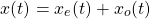• x(t): "original" signal
• xe(t): even part of x(t)
• xo(t): odd part of x(t)

### Periodic and Aperiodic

Signals are periodic when they repeat a specific pattern every time period T or sampling N.

Mathematically speaking, any signal that satisfies the following condition(s) is periodic: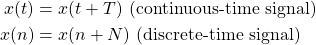### Energy and Power

A signal is a energy signal if it has finite energy, whilst a signal is a power signal if it has finite power.

Its worth noting that a signal cannot be both, energy and power simultaneously, and that it may be neither of them.

#### Energy

The energy of a signal is calculated using: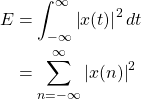#### Power

The power of a signal is calculated using: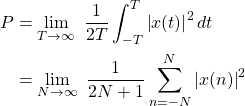### Real, Imaginary and Complex

Lastly, signals are also categorized as real and imaginary:

• Real: A signal is real when it has no imaginary part, meaning that the imaginary part is zero.
• Imaginary: A signal is imaginary when it has no real part, meaning that the real part is zero.
A signal where both parts (real and imaginary) are non-zero is considered complex.

An easy way to check if a signal is real or imaginary is using the complex conjugate of the signal: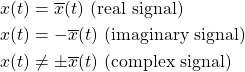## Basic Signal Types

### Unit Step Function

The unit step function, u(t), is defined as: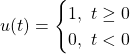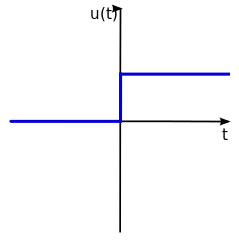[Image 2]

This function is the best test signal.

### Unit Impulse Function

The unit impulse function, δ(t), is defined as: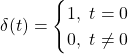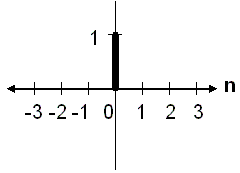[Image 3]

### Ramp Signal

The ramp signal, r(t), is defined as: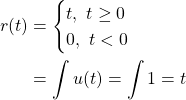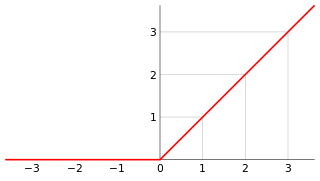[Image 4]

### Parabolic Signal

A parabolic signal t2/2can be easily defined using r(t) or u(t) as: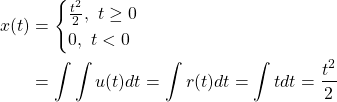### Signum Signal

Turning the unit step function into an odd function [u(t) = -u(-t)] creates the so called signum or sign function, sgn(x):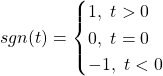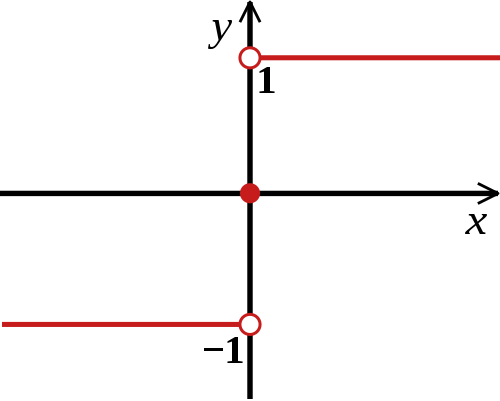[Image 5]

### Exponential Signal

Exponential signals are of the generic form: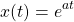The shape of the exponential depends on the value of the parameter a:

• a = 0 → e0 = 1
• a < 0 → decaying exponential
• a > 0 → raising exponential

### Sinusoidal Signal

Any signal of the form: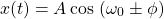or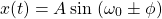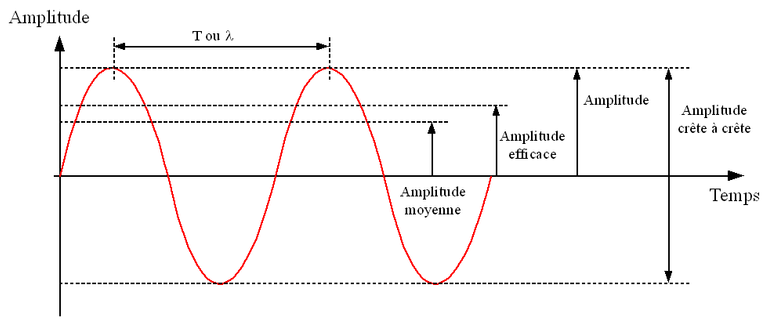[Image 6]

### Sinc and Sampling Functions

The sinc function, sinc(t), is defined as: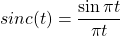The sampling function, sa(t), is defined as: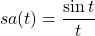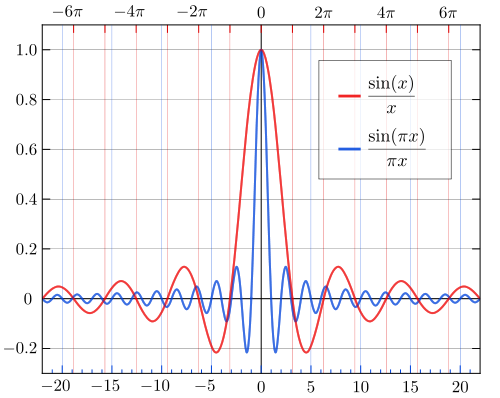[Image 7]

## RESOURCES:

### Images

Mathematical equations used in this article, where made using quicklatex.

## Final words | Next up

And this is actually it for today's post!

Next time we will dive even more into Signals...

See Ya!Keep on drifting!

Sort:Thanks for your contribution to the STEMsocial community. Feel free to join us on discord to get to know the rest of us!

Please consider supporting our funding proposal, approving our witness (@stem.witness) or delegating to the @stemsocial account (for some ROI).

Please consider using the STEMsocial app app and including @stemsocial as a beneficiary to get a stronger support.